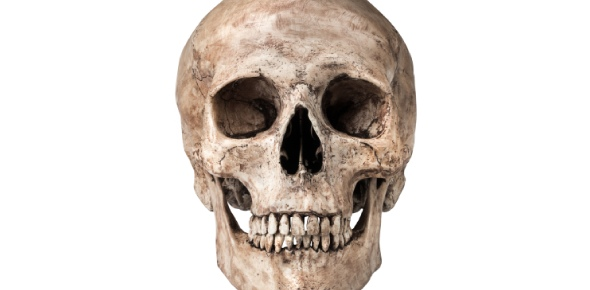# A Trivia Quiz On Skull Positioning!

20 Questions | Total Attempts: 2501SettingsCreate your own Quiz• 1.
What anatomical position would be used on a Towne method?
• A.

AP

• B.

PA

• 2.
An AP Towne using the IOML would use a tube angulation of____ degrees ______
• A.

37 degrees cephalic

• B.

30 degrees cephalic

• C.

37 degrees caudal

• D.

30 degrees caudal

• 3.
What must be visualized inside the foramen magnum of an AP Towne projection?
• A.

Mastoid

• B.

Dorsum sellae

• C.

Dens/ Odontoid process

• D.

Lamda

• 4.
On the lateral skull, the IOML is perpendicular to the____
• A.

IR

• B.

Front edge of the IR

• C.

Floor

• D.

Top edge of IR

• 5.
On a lateral skull, the centering is___ the EAM.
• A.

2 inches above

• B.

2 inches below

• C.

1 inch above

• D.

1 inch below

• 6.
On a PA Caldwell, where does the CR exit?
• A.

Nasion

• B.

Glabella

• C.

Acanthion

• D.

Mental point

• 7.
Where are the petrous ridges on a PA Caldwell with a 15 degree caudal angulation?
• A.

Lower 1/3 of orbits

• B.

Fully inside orbits

• C.

Above orbits

• D.

Below orbits

• 8.
What line is perpendicular to the IR in a PA skull with 0 degree angulation?
• A.

OML

• B.

IOML

• C.

MML

• D.

LDL

• 9.
The Dens/ Odontoid process is seen inside the foramen magnum on an SMV?
• A.

True

• B.

False

• 10.
On lateral fascial bones, the CR is centered to halfway between the outer cants and TEA
• A.

True

• B.

False

• 11.
Check all that are demonstrated on a waters view.
• A.

IOM's

• B.

Nasal septum

• C.

EAM

• D.

Mastoid processes

• 12.
Which line is perpendicular to the IR in a waters view?
• A.

MML

• B.

IOML

• C.

OML

• D.

GML

• 13.
In the modified Waters view, which line is perpendicular to the IR?
• A.

IOML

• B.

MSP

• C.

OML

• D.

AML

• 14.
Which facial reference line does not use the EAM?
• A.

GAL

• B.

MML

• C.

GOL

• D.

AML

• 15.
What Anatomy is demonstrated on a modified Towne view?
• A.

Zygomatic arches

• B.

Foramen magnum

• C.

Nasal septum

• D.

Orbits

• 16.
Which sinuses are visualized on a lateral sinus view?
• A.

Frontal sinuses

• B.

Ethmoid sinuses

• C.

Maxillary sinuses

• D.

Sphenoid sinuses

• 17.
On a PA Caldwell of the sinuses, the OML is tilted ______
• A.

15 degrees

• B.

30 degrees

• C.

20 degrees

• D.

10 degrees

• 18.
Where is the CR centered for an SMV of the skull?
• A.

1 1/2 inches below mandibular symphysis

• B.

2 inches below mandibular symphysis

• C.

1 1/2 inches below EAM

• D.

2 inches below EAM

• 19.
Where does the CR exit for a open mouth waters view?
• A.

Acanthion

• B.

Nasion

• C.

Glabella

• D.

LML

• 20.
On the open mouth waters view, the OML forms a ____ degree angle to the IR.
• A.

37

• B.

30

• C.

25

• D.

40

Related TopicsBack to top
×

Wait!
Here's an interesting quiz for you.